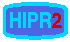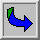Pixel Values

Each of the pixels that represents an image stored inside a computer has a pixel value which describes how bright that pixel is, and/or what color it should be. In the simplest case of binary images, the pixel value is a 1-bit number indicating either foreground or background. For a grayscale images, the pixel value is a single number that represents the brightness of the pixel. The most commonpixel format is the byte image, where this number is stored as an 8-bit integer giving a range of possible values from 0 to 255. Typically zero is taken to be black, and 255 is taken to be white. Values in between make up the different shades of gray.

To represent color images, separate red, green and blue components must be specified for each pixel (assuming an RGB colorspace), and so the pixel `value' is actually a vector of three numbers. Often the three different components are stored as three separate `grayscale' images known as color planes (one for each of red, green and blue), which have to be recombined when displaying or processing.

Multi-spectral images can contain even more than three components for each pixel, and by extension these are stored in the same kind of way, as a vector pixel value, or as separate color planes.

The actual grayscale or color component intensities for each pixel may not actually be stored explicitly. Often, all that is stored for each pixel is an index into a colormap in which the actual intensity or colors can be looked up.

Although simple 8-bit integers or vectors of 8-bit integers are the most common sorts of pixel values used, some image formats support different types of value, for instance 32-bit signed integers or floating point values. Such values are extremely useful in image processing as they allow processing to be carried out on the image where the resulting pixel values are not necessarily 8-bit integers. If this approach is used then it is usually necessary to set up a colormap which relates particular ranges of pixel values to particular displayed colors.